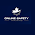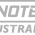## SAT Physics Thermodynamics - Internal Energy

INTERNAL ENERGY
Internal energy, U, is the total energy of a system. Adding up all possible energies that comprise the total energy of a system would be extremely complex. However, doing this is not necessary.   Thermodynamics is not concerned with the actual value of internal energy, U, but rather with the change in internal energy, ΔU. It is the change in internal energy that makes engines operate. In thermodynamics, all but one of the energies comprising internal energy are held constant. Thermal energy, Eth or Ethermal, is the only energy that is changing. The change in all other energies will be equal to zero.
ΔU= ΔEthermal
The thermal energy of a gas is the sum of all the microscopic kinetic energies of the randomly moving gas particles. This energy can be calculated and results in the following equation for the change in internal energy of a gas:It is unlikely that this equation will appear on the exam. However, it has been included here to show the important direct relationship between temperature change and the change in internal energy. In addition, the sign on the change in internal energy in this equation is the key to setting the correct sign on other thermodynamic variables.

During a thermodynamic process there are three possibilities for the value of internal energy.
1. INTERNAL ENERGY INCREASES. If energy is added to the system, the internal energy increases, +ΔU.
This is accompanied by an increase in the temperature of the system, +ΔT.
2. INTERNAL ENERGY DECREASES. If energy is removed from the system, the internal energy decreases, -ΔU.
This is accompanied by a decrease in the temperature of the system, -ΔT.
3. ISOTHERMAL PROCESS. This is a thermodynamic process where the temperature remains constant, ΔT = 0.
If the temperature is not changing, then the internal energy is not changing, ΔU=0.
ENERGY TRANSFER IN THERMODYNAMICS
To visualize a thermodynamic system, think of a gas trapped in a cylinder with a movable piston. Only the gas particles constitute the system. The cylinder and piston are part of the environment. They simply contain the gas in an adjustable and measurable volume. This system is shown in Figure 19.1.Figure 19.1. A thermodynamic system

Work and heat are the two processes that can transfer energy into and out of the gas system shown in Figure 19.1. Work and heat are in units of joules. Work and heat are the changes to state functions, such as internal energy U, kinetic energy K, and the energy of a spring Us.

Work
Work, W, is a macroscopic (visible) transfer of energy involving a net force, ΣF, acting through a distance, d. Work is a mechanical way to add or remove energy from the gas system by physically moving the piston. The force that moves the piston is created by pressure acting on the inside and outside areas of the piston. The pressure of the gas inside the cylinder pushes the piston outward. Forces from the environment, such as the pressure of the atmosphere surrounding the cylinder, push the piston inward.
Moving the piston definitely involves a volume change, AV; but what about pressure? Under some conditions, the pressure of the gas changes when work moves the piston. Under special conditions, the pressure remains constant as the piston moves. This is known as an isobaric process. Under these special conditions, work is a product of the constant pressure and changing volume.
W= - PΔV
Whether the pressure of the gas is changing or is constant, there are three possible outcomes. Which particular outcome occurs depends on how the magnitudes of the pressures inside and outside the cylinder compare to one another.
1. WORK DONE ON THE GAS. If the pressure outside the cylinder is greater than the pressure inside the cylinder, then work is done on the gas while moving the piston inward. The volume of the gas is compressed, -ΔV The environment adds energy to the system, increasing the internal energy, +ΔU. Work is a change in energy. The work done on the gas is positive, + W, increasing the internal energy of the system.
2. WORK DONE BY THE GAS. If the pressure inside the cylinder is greater than the pressure outside the cylinder, then work is done by the gas while moving the piston outward. The volume of the gas expands, +ΔV In the process, the gas uses its energy to push the piston outward, which causes internal energy to decrease, -ΔU. Therefore, the work done by the gas is negative, -W.
3. ISOMETRIC (ISOCHORIC) PROCESS. This is a thermodynamic process where the volume of the gas remains constant. This occurs when the pressure of the gas system inside the cylinder is the same as the pressure of the environment outside the cylinder. The net force is zero. The piston cannot move, ΔV= 0, and no work is done, W= 0.
Heat
As discussed in the previous chapter, heat (Q), is a microscopic (invisible) transfer of thermal energy between objects having different temperatures. Heat can be added or removed by touching a heat reservoir to the cylinder. An example of a heat reservoir could be air or water surrounding the cylinder. The energy transferred into or out of the reservoir is negligible compared with the huge size of the reservoir. As a result, heat reservoirs have constant temperature and transfer heat at a constant rate. A hot reservoir has a temperature greater than the gas in the cylinder. A cold reservoir has a temperature lower than the gas in the cylinder.
Heat flows from high temperature to low temperature. There are three possible outcomes for heat transfer depending on the temperature of the heat reservoir as compared with the temperature of the trapped gas in the cylinder.
1. HEAT ADDED. If the heat reservoir has a higher temperature (hot reservoir) than the gas inside the cylinder, heat flows from the reservoir into the cylinder. Internal energy increases, +ΔU. Therefore, heat added is considered positive, +Q.
2. HEAT REMOVED. If the heat reservoir has a lower temperature (cold reservoir) than the trapped gas inside the cylinder, heat flows out of the cylinder into the reservoir. Internal energy decreases, - ΔU Therefore, heat removed is considered negative, -Q.
3. ADIABATIC PROCESS. This is a thermodynamic process where no heat is added or removed. This occurs when the heat reservoir and the trapped gas are in thermal equilibrium (same temperature). Heat transfer requires a temperature difference. So no net heat flow occurs between objects in thermal equilibrium, Q = 0.
ENERGY MODEL SUMMARIZED
Figure 19.2 summarizes the interactions among internal energy, work, and heat. The signs on each variable are linked to their effect on the internal energy, ΔU, of the system (the gas trapped in the cylinder). Table 19.2 summarizes additional information and trends.Figure 19.2. Thermodynamics energy model

Table 19.2 Interactions Among Internal Energy, Work, and HeatFIRST LAW OF THERMODYNAMICS
The first law of thermodynamics is a statement of conservation of energy for thermal processes. Work, W, and heat, Q, are the only two processes that can affect the internal energy, U, of a gas. Their effect can be summarized as follows.
• For a system where internal energy is composed of only thermal energy, the change in internal energy of the system is equal to the energy transferred into or out of the system by work and heat.
• The previous statement can be summarized as an equation:
ΔU=Q+W
Examples 19.1 and 19.2 demonstrate how the first law of thermodynamics is applied.

First Law of Thermodynamics
During a thermodynamic process, 200 joules of heat are added to a gas while 300 joules of work are done by the gas. Determine the change in internal energy.

WHAT'S THE TRICK?

Apply the first law of thermodynamics. Heat added is positive, +Q. Work done by the gas pushes the piston outward, expanding the volume. To accomplish this, the gas loses energy and work is negative, - W.
ΔU = Q+W
ΔU = (200 J) + (-300 J)
ΔU = - 100 J

First Law of Thermodynamics
(A) During an isothermal process, 1,000 joules of heat are removed from a trapped gas. Determine the change in internal energy of the gas

WHAT'S THE TRICK?

You need to know the definition of the word “isothermal.” In an isothermal process, the temperature remains constant,
ΔT = 0, and the internal energy does not change, ΔU = 0.
(B) Determine the work done on or by the gas.

WHAT'S THE TRICK?

Apply the first law of thermodynamics, including the information in part A, ΔU = 0. The heat removed is negative, -Q.
ΔU = Q + W
(0) = (-1,000 J) + W
W = 1,000 J
(C) Is this work done on the gas or by the gas?

WHAT'S THE TRICK?

You need to know the difference between work done on the gas and work done by the gas. Positive work means that the internal energy of the gas is increasing. The environment must compress the gas in order to increase the internal energy. This is work done on the gas.

1.Thanks for sharing such a nice article. It is really useful to me and all people. One thing in my mind came while reading that it is not wasting of time. currency exchange app shopify , currency converter box free online , best shopify apps to boost sales in 2019

2.I guess you are a smart cookie because of your knowledge and insight. The articles brings some advice. I learn more from them. Please update more posts. I usually read them. Thank you very much.sale discount app on Shopify , Product discount master , best shopify free apps to boost sales in 2019

3.Extremely useful information which you have shared here.propane cylinder handing course This is a great way to enhance knowledge for us, and also helpful for us. Thankful to you for sharing an article like this.

4.Thanks for sharing the best information and suggestions, it is very nice and very useful to us. I appreciate the work that you have shared in this post. Keep sharing these types of articles here. Thermal Imaging Monocular